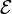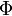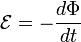# Weber (unit)

The weber (symbol Wb) is the SI unit of magnetic flux. It is named for the German physicist Wilhelm Eduard Weber.

The ninth Conférence Générale des Poids et Mesures (General Conference on Weights and Measures) ratified in 1948 a definition of weber based on Faraday's law for magnetic induction. Faraday's law connects electromotive force$\mathcal{E}$ (in volt) to the rate of change of magnetic flux$\Phi\,$ (in Wb/s) through one loop of conducting wire (a "turn"):$\mathcal{E} = -\frac{d\Phi}{dt}$

If the magnetic flux Φ is linear in time—Φ changes with uniform rate—and if Φ = 0 at t = 0, then it follows that$\Phi(t) = - t\, \mathcal{E}.$

This equation forms the basis of the formal definition, which reads:

One weber is the magnetic flux which, linking a circuit of one turn, would produce in it an electromotive force of 1 volt if it were reduced to zero at a uniform rate in 1 second. (Resolution 2, International Committee for Weights and Measures. 1946).

Dimension: Wb = V⋅s = N⋅m⋅A−1 = kg⋅A−1⋅s−2⋅m2 = T⋅m2, where A stands for ampere, T for tesla, V for volt, and N for newton.

## Reference

1. BIPM brochure about SI. PDF page 52; paper page 144.